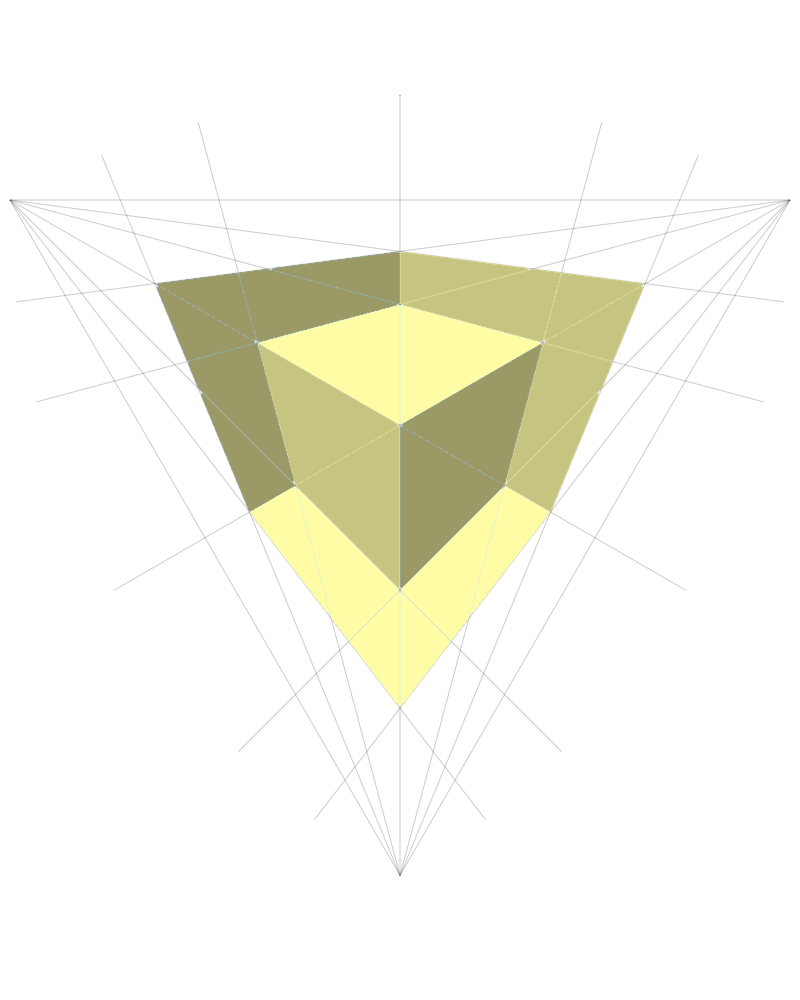# Triangle

I wanted to draw a triangle, so I sat down on the ground and thought about it for a while.

When it all comes down to it. What it is, you see. How it works. Well a triangle has. It has 3.

• 3 points.
• 3 corners.
• 3 angles.

It's 3 lines really.

So I want to draw 3 lines.

The lines are all the same size.

An equal triangle. An equilateral triangle.

(Are the lines the same because the angles are the same or are the angles the same because the lines are the same? It's a trickly-pickly question and you might think that both are true—but one of these is the MOST true—and you can work it out if you consider shapes with more than 3 sides)

So I'll have:

Draw a line

Draw a line

Draw a line

...but I need something in between.

I need to turn the paper around.

Or if I can't turn the paper, I could turn myself.

I imagine I am a turtle. And for a moment I wonder if I have always been a turtle. Perhaps I have just been a turtle pretending to be a human boy. At last: I am turtle again. And I speak the language of turtle.

I will draw a line. Length 200. A good length for a line. I will draw 3. And I will turn myself around in between each line. (The paper will not move)

Same thing is repeated three times.

I picked the angle of 120° because... well I've played this game before.

I know if we are drawing a shape with 3 sides then we get a circle, of 360°, and divide it into 3 equal turns.

``````360/3 = 120
``````

If we were drawing a shape with 36 equal sides we would have:

``````360/36 = 10
``````

I should make the looping more obvious.

Now I want to draw something fun with this triangle.

I have read something about drawing the mid point on each of the lines and joining it up to something.

I make a function, mark, that scratches a little line at the mid-point of each line.

What I want to do is join the midpoint to the opposite corner.

I have a way of doing that.

Here's what it looks like with some of the areas shaded: